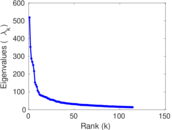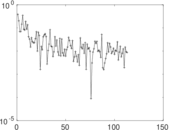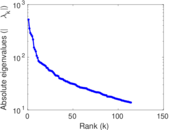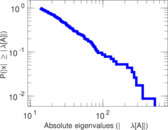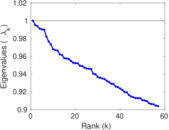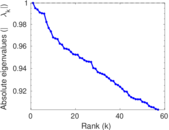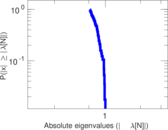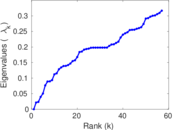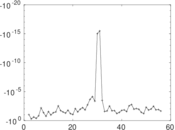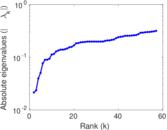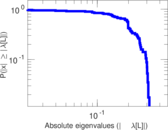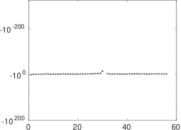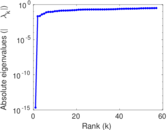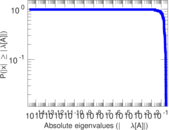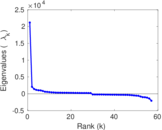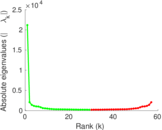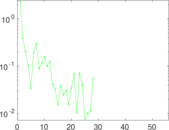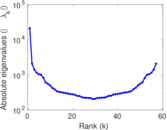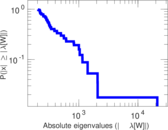# Wikiquote edits (ja)

This is the bipartite edit network of the Japanese Wikisource. It contains users and pages from the Japanese Wikisource, connected by edit events. Each edge represents an edit. The dataset includes the timestamp of each edit.

 Code `qja` Internal name `edit-jawikisource` Name Wikiquote edits (ja) Data source http://dumps.wikimedia.org/ AvailabilityDataset is available for download Consistency checkDataset passed all tests Category Authorship network Dataset timestamp 2017-10-20 Node meaning User, article Edge meaning Edit Network formatBipartite, undirected Edge typeUnweighted, multiple edges Temporal dataEdges are annotated with timestamps

## Statistics

 Size n = 21,208 Left size n1 = 1,143 Right size n2 = 20,065 Volume m = 71,279 Unique edge count m̿ = 36,930 Wedge count s = 40,781,909 Claw count z = 64,488,942,393 Cross count x = 88,566,016,043,107 Square count q = 3,752,705 4-Tour count T4 = 193,251,204 Maximum degree dmax = 10,627 Maximum left degree d1max = 10,627 Maximum right degree d2max = 704 Average degree d = 6.721 90 Average left degree d1 = 62.361 3 Average right degree d2 = 3.552 40 Fill p = 0.001 610 25 Average edge multiplicity m̃ = 1.930 11 Size of LCC N = 20,493 Diameter δ = 13 50-Percentile effective diameter δ0.5 = 3.404 75 90-Percentile effective diameter δ0.9 = 5.014 77 Median distance δM = 4 Mean distance δm = 3.789 70 Gini coefficient G = 0.754 825 Balanced inequality ratio P = 0.204 134 Left balanced inequality ratio P1 = 0.086 224 6 Right balanced inequality ratio P2 = 0.298 714 Relative edge distribution entropy Her = 0.755 881 Power law exponent γ = 3.305 71 Tail power law exponent γt = 2.761 00 Tail power law exponent with p γ3 = 2.761 00 p-value p = 0.000 00 Left tail power law exponent with p γ3,1 = 1.641 00 Left p-value p1 = 0.521 000 Right tail power law exponent with p γ3,2 = 3.881 00 Right p-value p2 = 0.099 000 0 Degree assortativity ρ = −0.139 585 Degree assortativity p-value pρ = 4.920 71 × 10−160 Spectral norm α = 518.662 Algebraic connectivity a = 0.021 428 7 Spectral separation |λ1[A] / λ2[A]| = 1.471 79 Controllability C = 19,038 Relative controllability Cr = 0.900 397

## Plots

### Fruchterman–Reingold graph drawing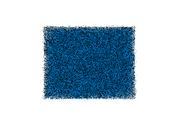### Degree distribution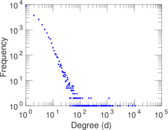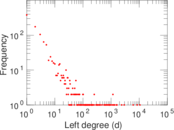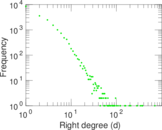### Cumulative degree distribution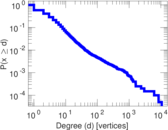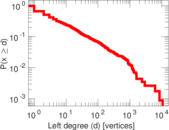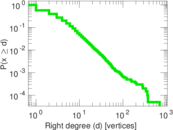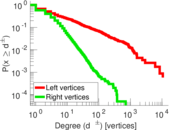### Lorenz curve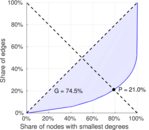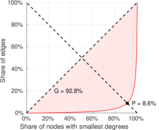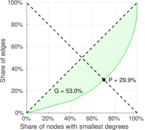### Spectral distribution of the adjacency matrix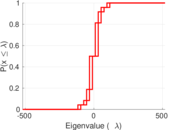### Spectral distribution of the normalized adjacency matrix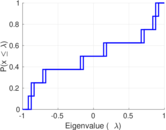### Spectral distribution of the Laplacian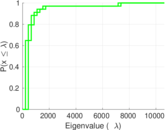### Spectral graph drawing based on the adjacency matrix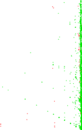### Spectral graph drawing based on the Laplacian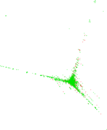### Spectral graph drawing based on the normalized adjacency matrix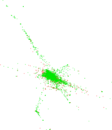### Degree assortativity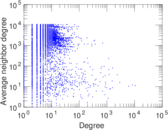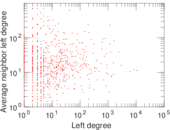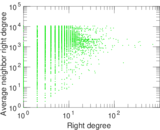### Zipf plot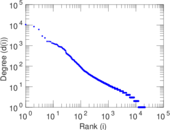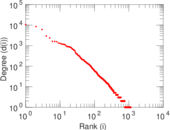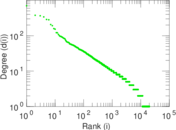### Hop distribution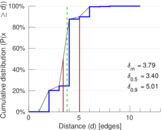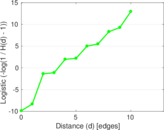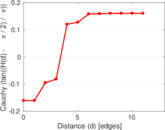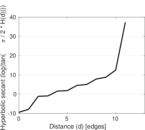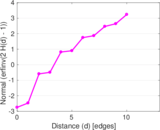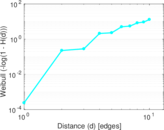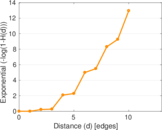### Double Laplacian graph drawing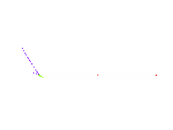### Delaunay graph drawing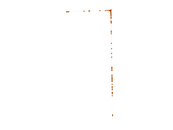### Edge weight/multiplicity distribution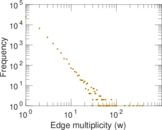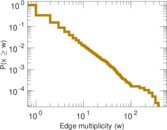### Temporal distribution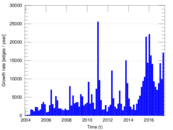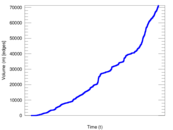### Temporal hop distribution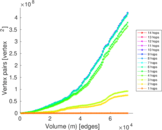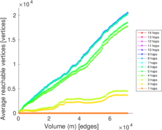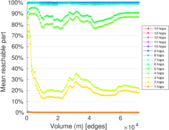### Diameter/density evolution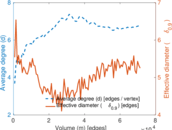### Matrix decompositions plots